# Derivatives definition and derivatives of various functions What

• Slides: 32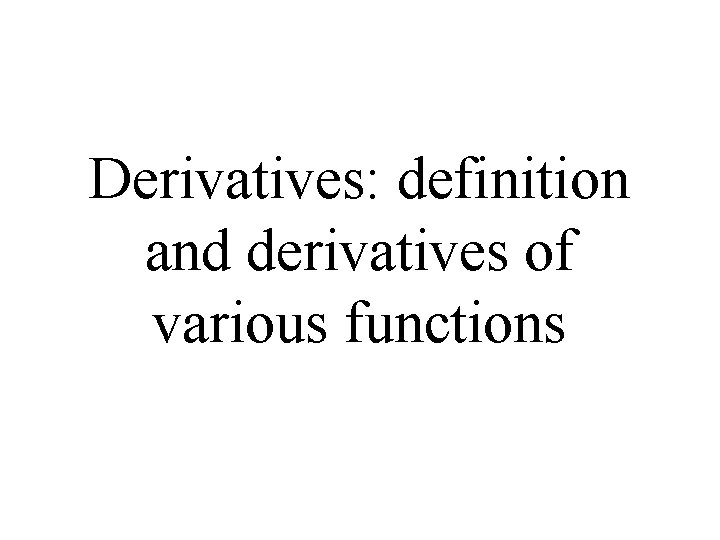Derivatives: definition and derivatives of various functions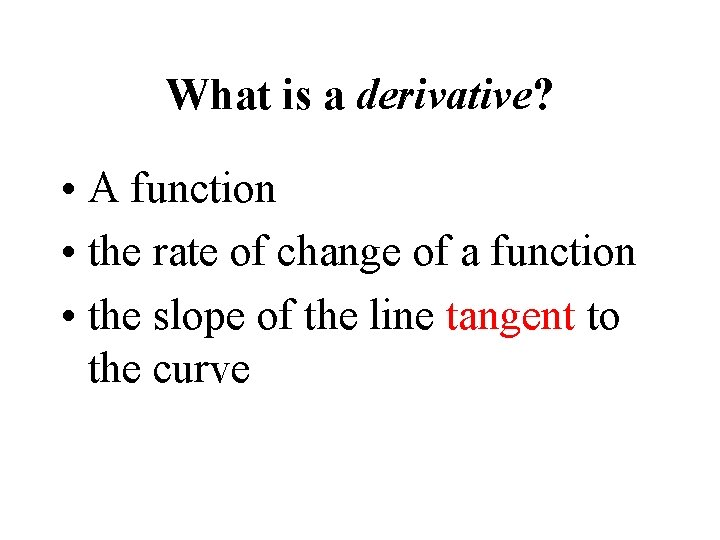What is a derivative? • A function • the rate of change of a function • the slope of the line tangent to the curveThe tangent line single point of intersection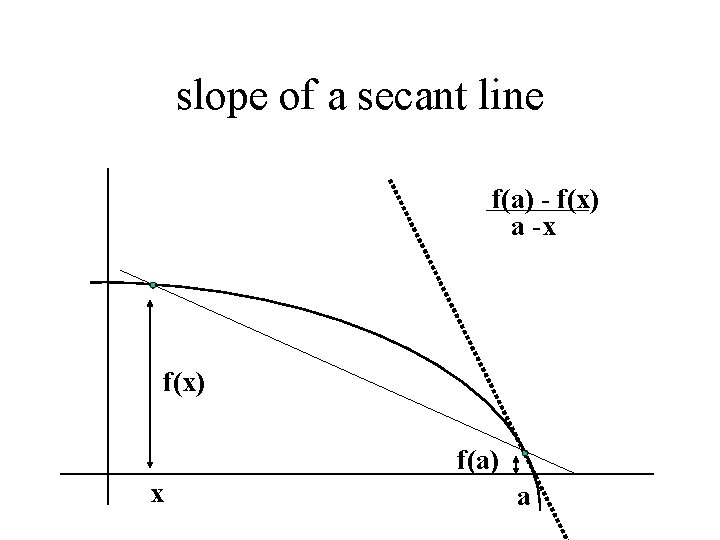slope of a secant line f(a) - f(x) a -x f(x) f(a) x a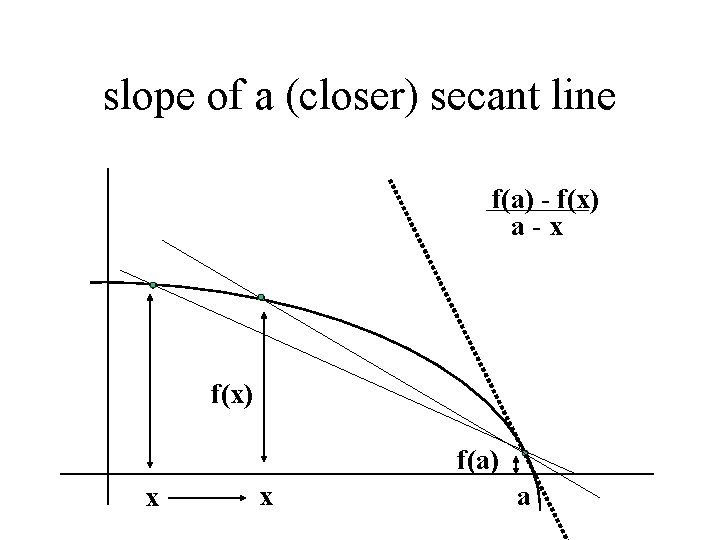slope of a (closer) secant line f(a) - f(x) a-x f(x) f(a) x x acloser and closer… a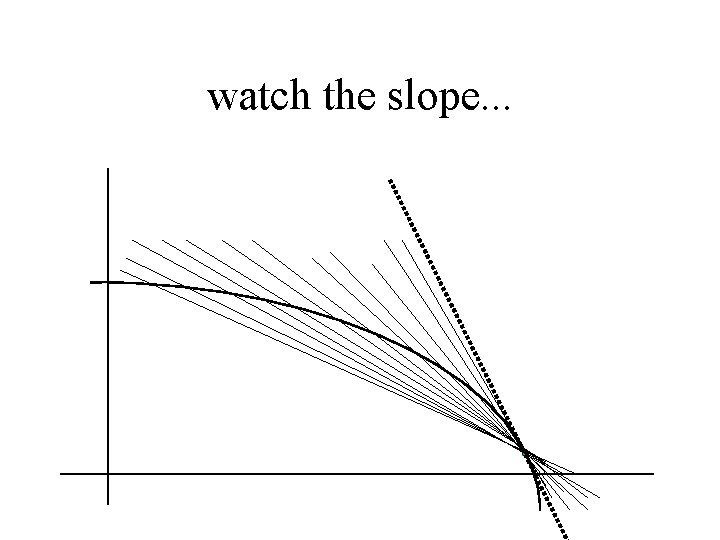watch the slope. . .The slope of the secant line gets closer and closer to the slope of the tangent line. . .As the values of x get closer and closer to a! x a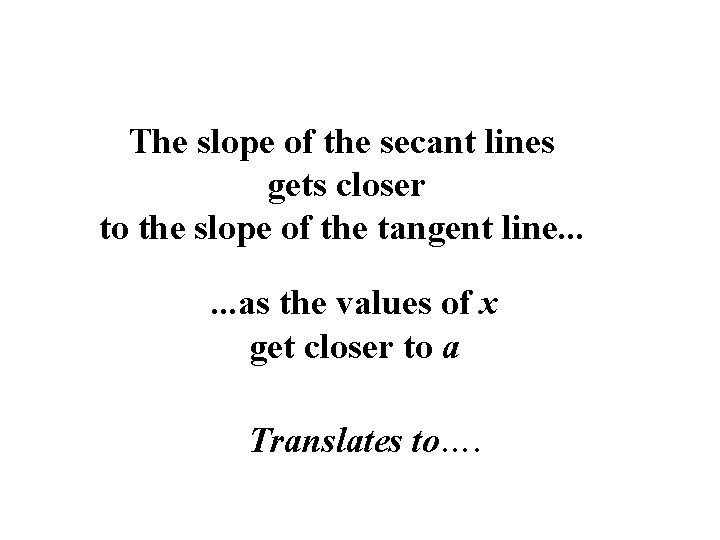The slope of the secant lines gets closer to the slope of the tangent line. . . as the values of x get closer to a Translates to….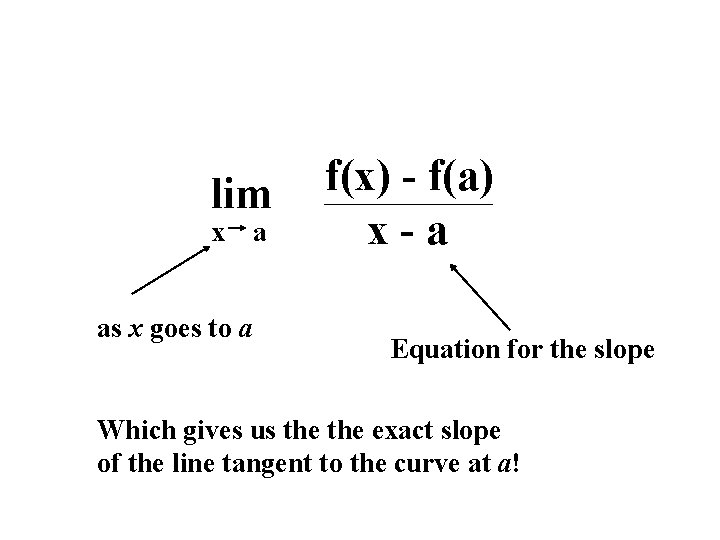lim x as x goes to a a f(x) - f(a) x-a Equation for the slope Which gives us the exact slope of the line tangent to the curve at a!The tangent line problem secant line (x, f(x)) is the point of tangency and f(x+ x ) – f(x) is a second point on the graph of f.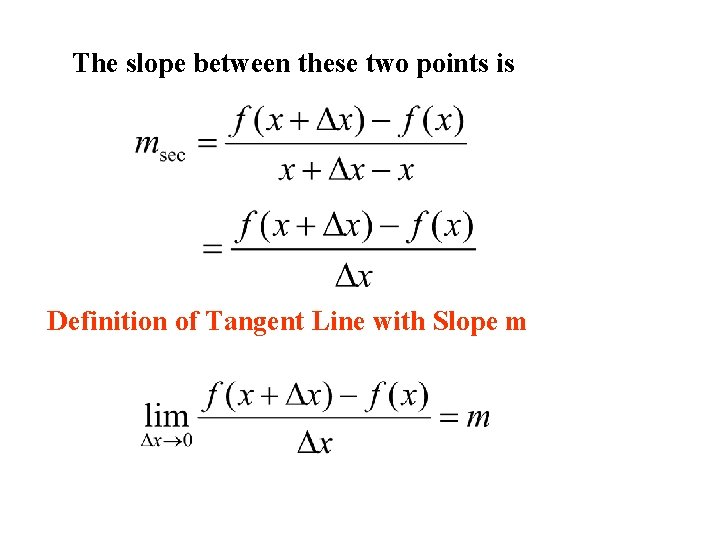The slope between these two points is Definition of Tangent Line with Slope m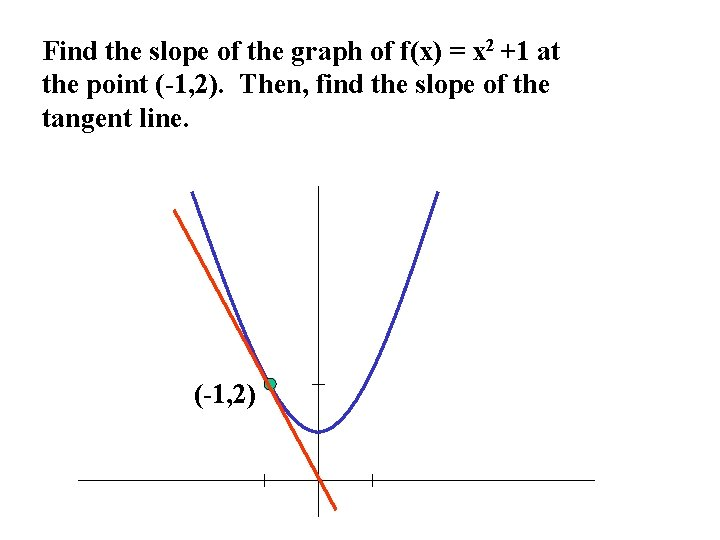Find the slope of the graph of f(x) = x 2 +1 at the point (-1, 2). Then, find the slope of the tangent line. (-1, 2)f(x) = x 2 + 1 Therefore, the slope at any point (x, f(x)) is given by m = 2 x What is the slope at the point (-1, 2)? m = -2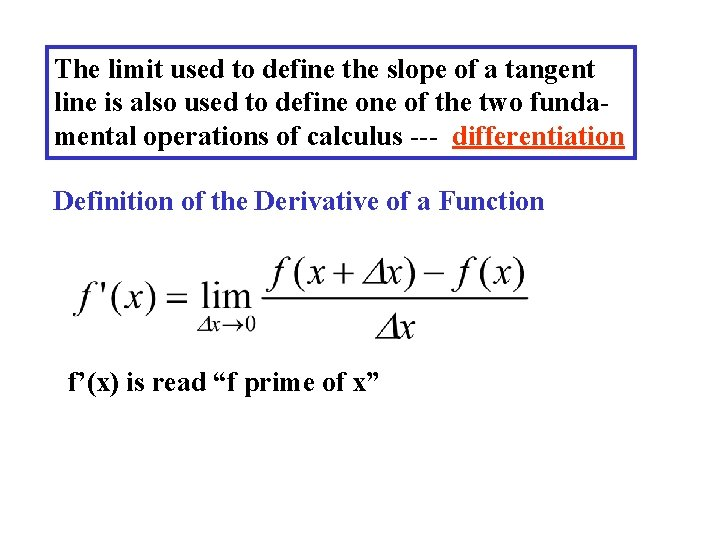The limit used to define the slope of a tangent line is also used to define of the two fundamental operations of calculus --- differentiation Definition of the Derivative of a Function f’(x) is read “f prime of x”Find f’(x) for f(x) = and use the result to find the slope of the graph of f at the points (1, 1) & (4, 2). What happens at the point (0, 0)? 1Derivative of a Function “the derivative of f with respect to x” “y prime” “the derivative of y with respect to x” “the derivative of f of x”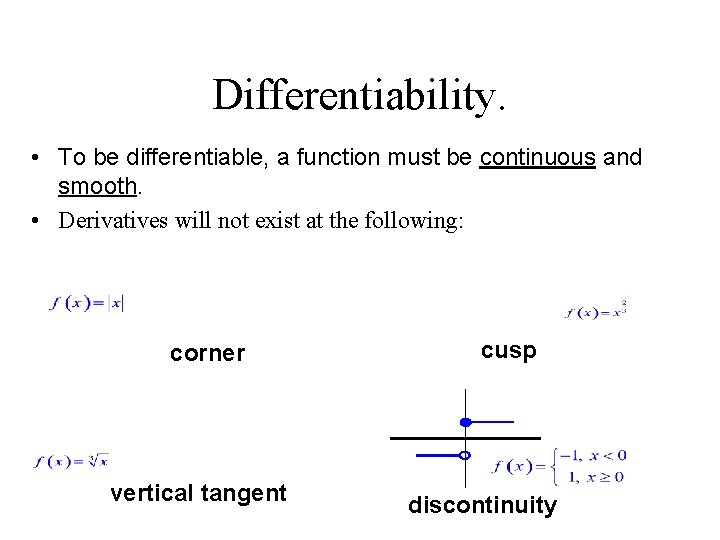Differentiability. • To be differentiable, a function must be continuous and smooth. • Derivatives will not exist at the following: corner vertical tangent cusp discontinuity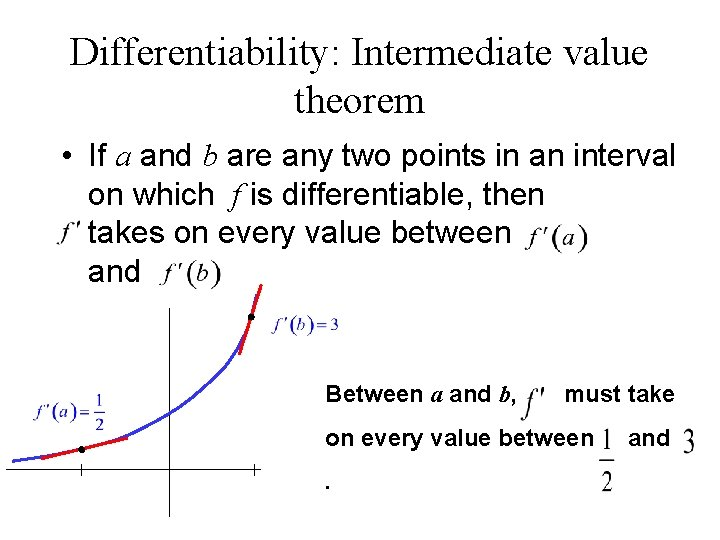Differentiability: Intermediate value theorem • If a and b are any two points in an interval on which f is differentiable, then takes on every value between and Between a and b, must take on every value between. and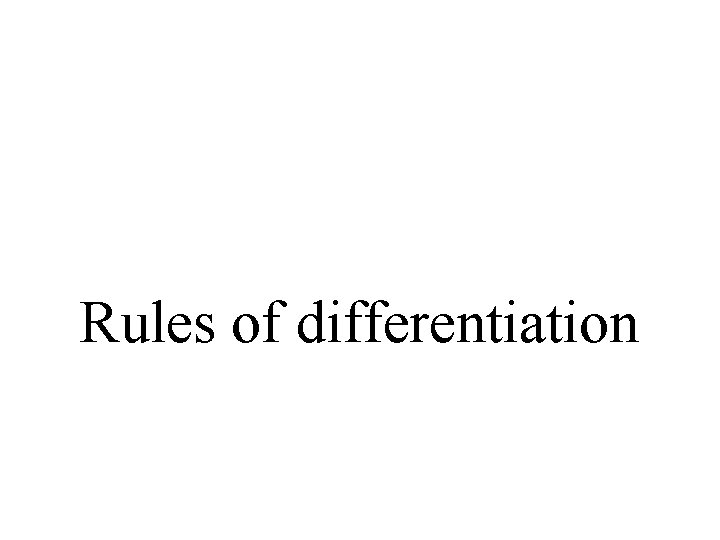Rules of differentiation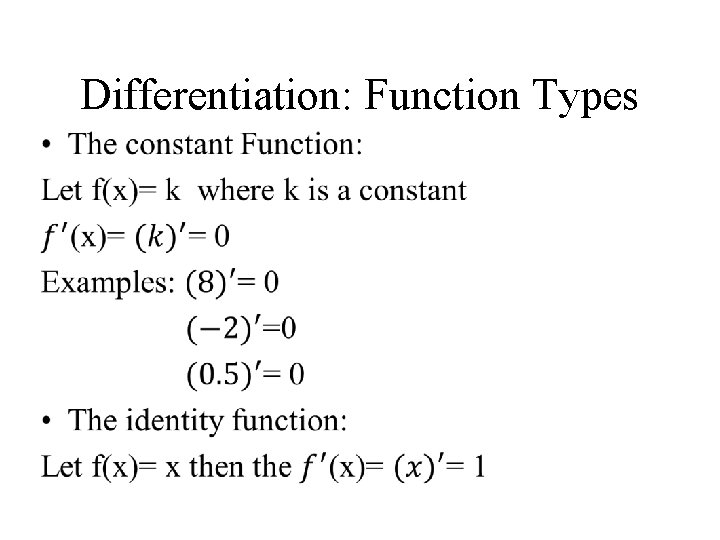Differentiation: Function Types •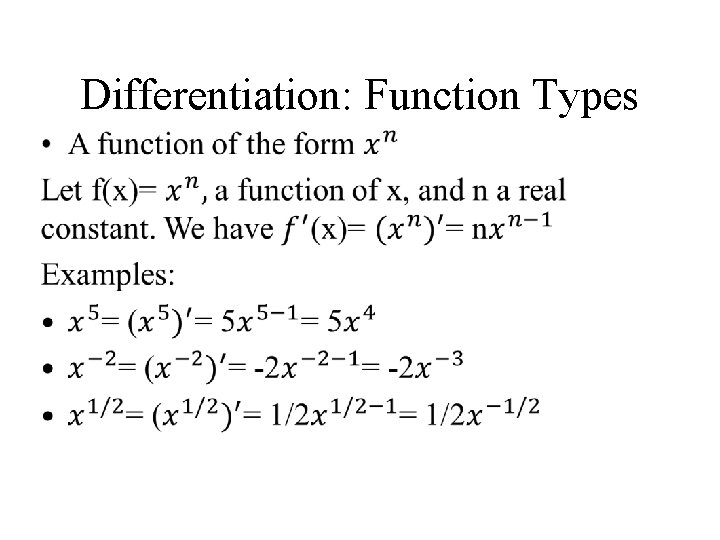Differentiation: Function Types •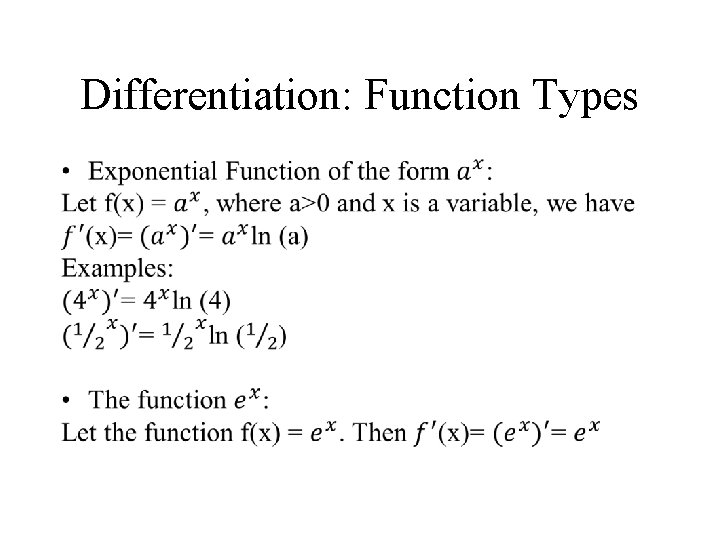Differentiation: Function Types •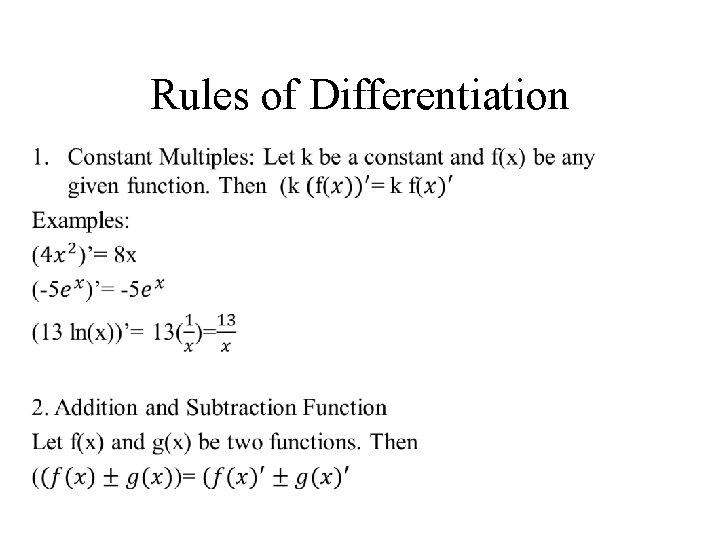Rules of Differentiation •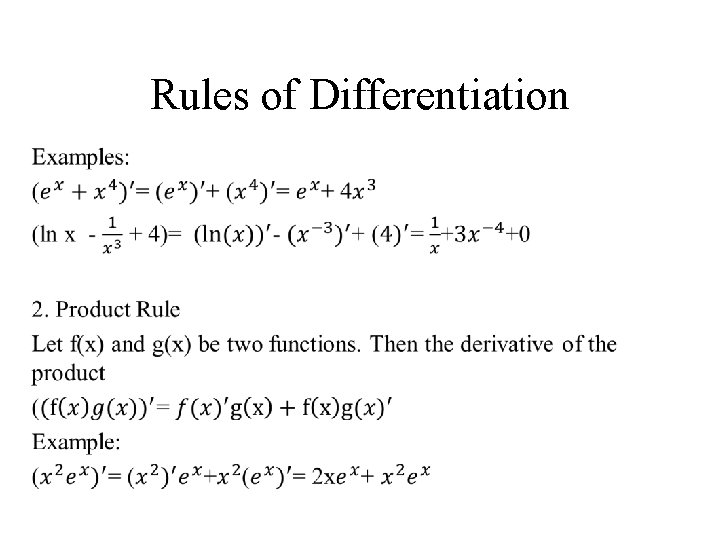Rules of Differentiation •Rules of Differentiation •Rules of Differentiation •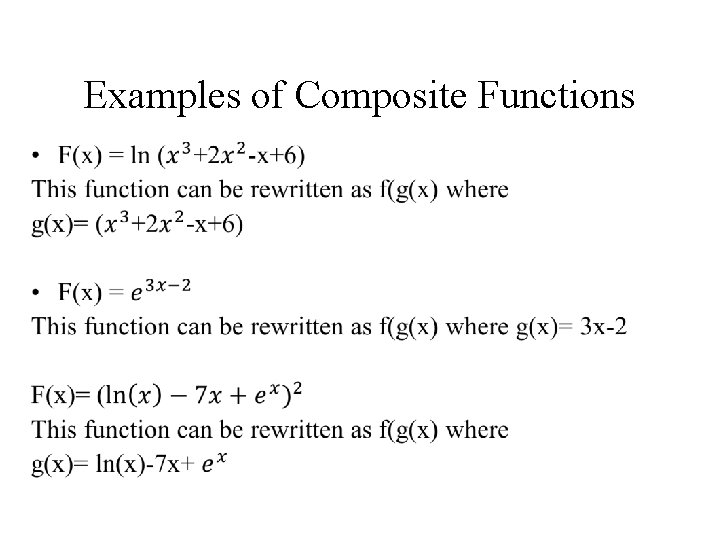Examples of Composite Functions •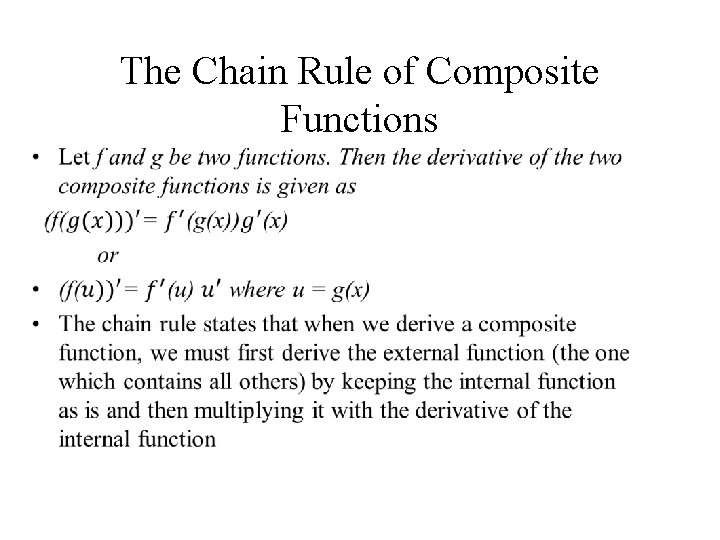The Chain Rule of Composite Functions •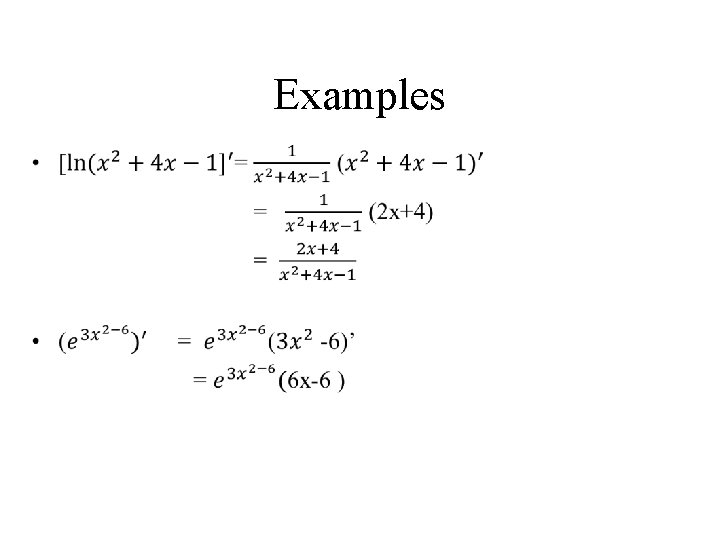Examples •Session Problem Set •